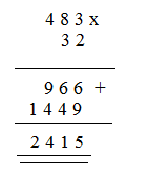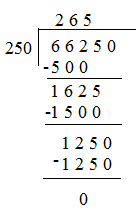# Word Problems on Number Operations

A number is a figure or a value to denote the count or quantity of something. The counting numbers or natural numbers include the numerals 1, 2, 3, 4, etc and are the fundamental of a number. Arithmetic is the elementary branch which studies about numbers, their properties, and arithmetical operations. There are basically four operations- additions (+), subtraction (-), multiplication($\times$) and division ($\div$). Each operation helps us to calculate how more or less an object than the other. Let’s practice how to solve word problems using these arithmetical operations.

## Word Problems Examples

Example 1: Akshit won a lottery of cash prize Rs.157800. He deposited the amount in his bank account. If he had Rs.45000 in his account, what is his current bank balance?Solution: We have to find the total amount in Akshit’s bank account. Given,

Amount of cash Akshit won by lottery = Rs.157800

Amount Akshit had before winning lottery= Rs.45000

Total amount Akshit have after winning lottery= 157800 + 45000

$157800+\frac{45000}{202800}$

Akshit have Rs.202800 as his current balance.

Example 2: Babuji bought a car for Rs. 375000. After three years he sold his car and bought new car for Rs. 830000. How much more has Babuji paid for his new car?

Solution: We have to find the price difference. Given,

Cost of old car= Rs. 375000

Cost of new car= Rs. 830000

Price difference between new car and old car = 830000 – 375000

$830000+\frac{375000}{455000}$<

Babuji has paid Rs. 455000 more for his new car.

Example 3: Prateek is a bookworm. He has a home library and he arranges 483 books per shelves. If there are 32 shelves like this, what will be the count of books in Prateek’s library?Solution: Given,

Total no. of shelves = 32

No. of books per shelve = 483

Total no. of books in the library = 483 x 32Prateek has 2415 books in his library.

Example 4: A shoe factory manufactured 66250 shoes in 250 days. How many shoes did it manufacture per day?

Solution: Given,

Total no. of shoes manufactured= 66250

Total no. of days taken to manufacture 66250 shoes = 250 days

No. of shoes manufactured per day= 66250 ÷ 250Hence, factory produced 265 shoes per day.

To solve more word problems on arithmetical operations, download Byju’s – The Learning App from Google Play Store and watch interactive videos. Also, take free tests to practice for exams.